# RS Aggarwal Solutions for Class 12 Chapter 13: Methods of Integration Exercise 13A

Integration by Substitution is the main concept which is covered under Exercise 13A of Chapter 13. The problems are solved by teachers having a high knowledge of concepts with the aim of helping students score well in the board exam. The solved examples before each exercise give an idea to the students about the kind of questions that would appear in the exam. Students who aspire to perform well in the board exam can download RS Aggarwal Solutions Class 12 Maths Chapter 13 Methods of Integration Exercise 13A PDF using links, which are provided below.

## RS Aggarwal Solutions for Class 12 Maths Chapter 13: Methods of Integration Exercise 13A Download PDF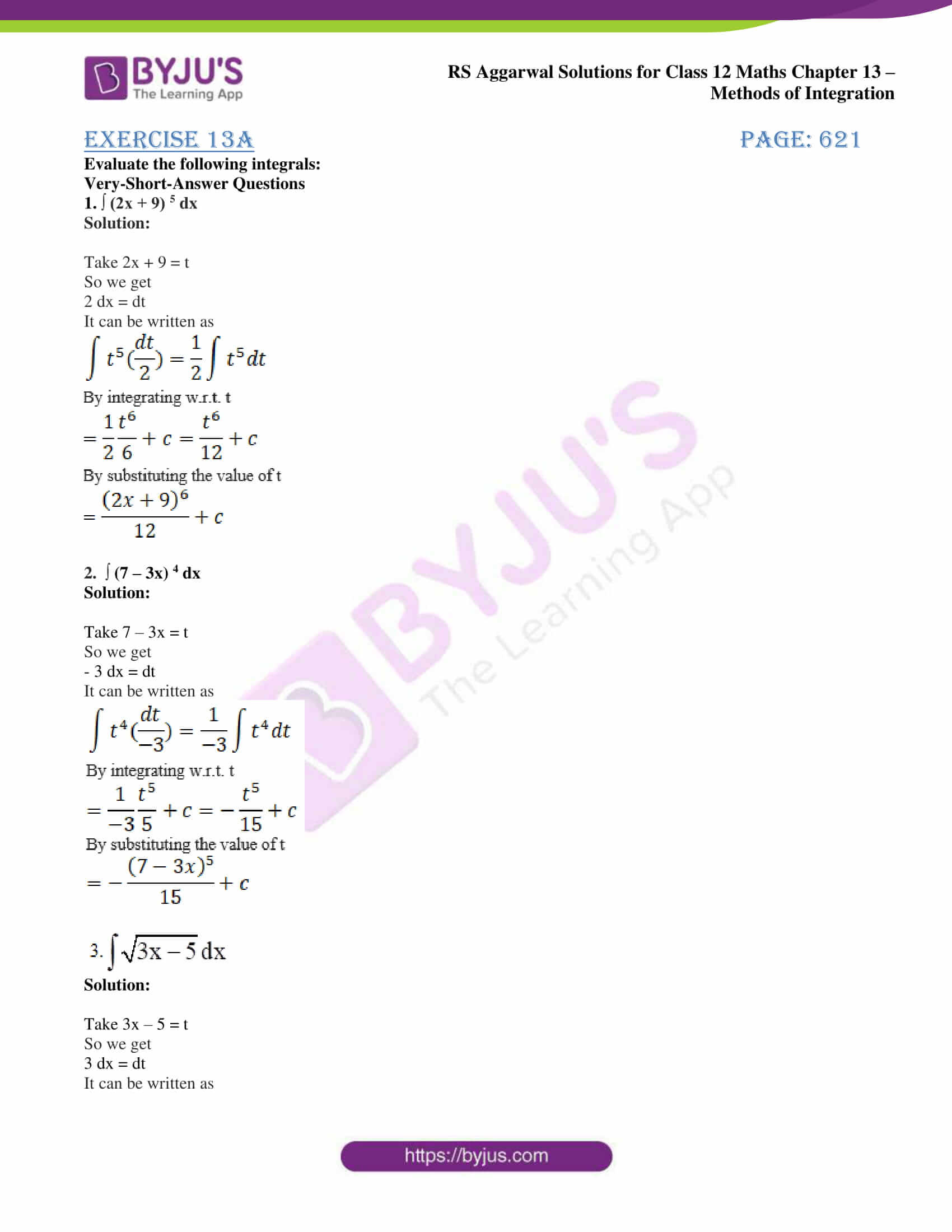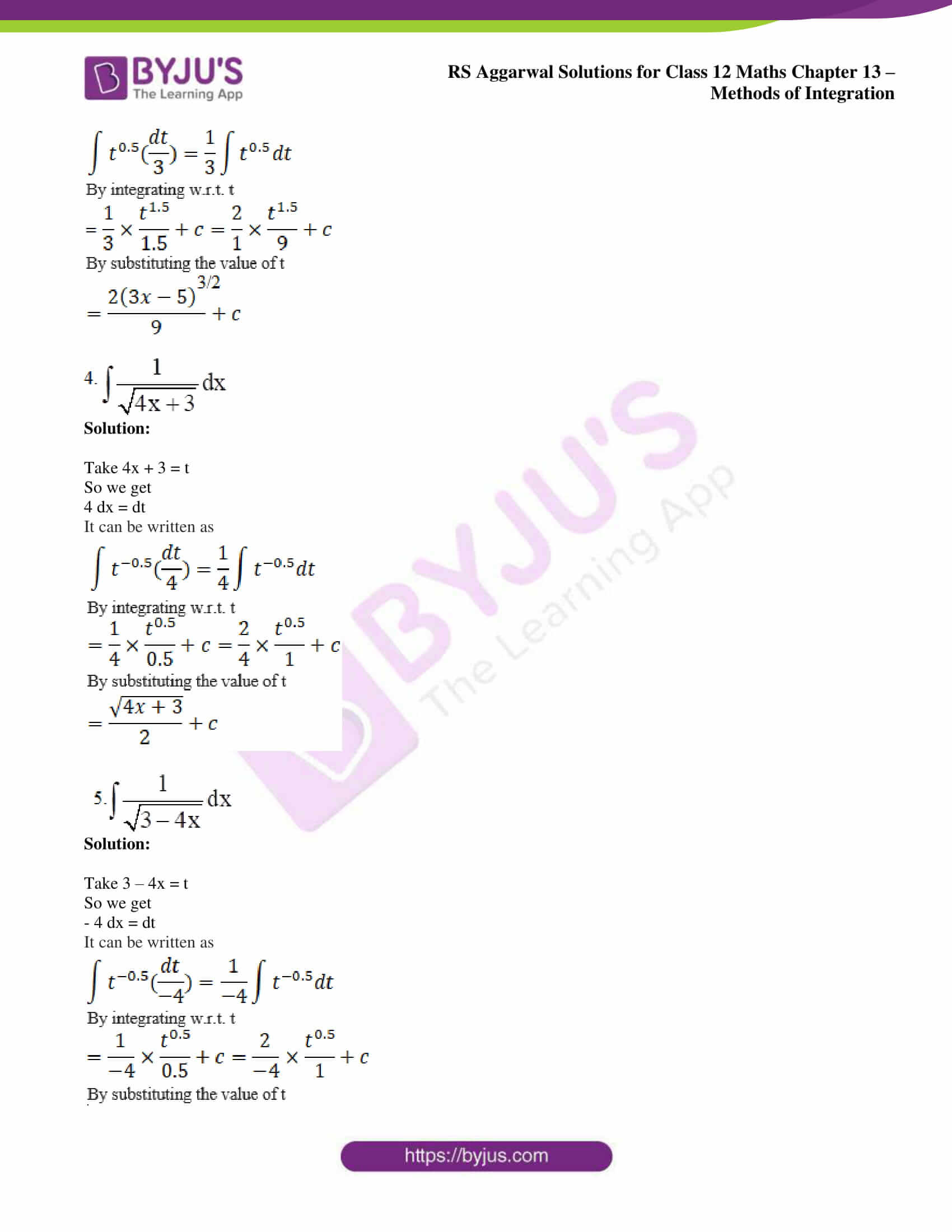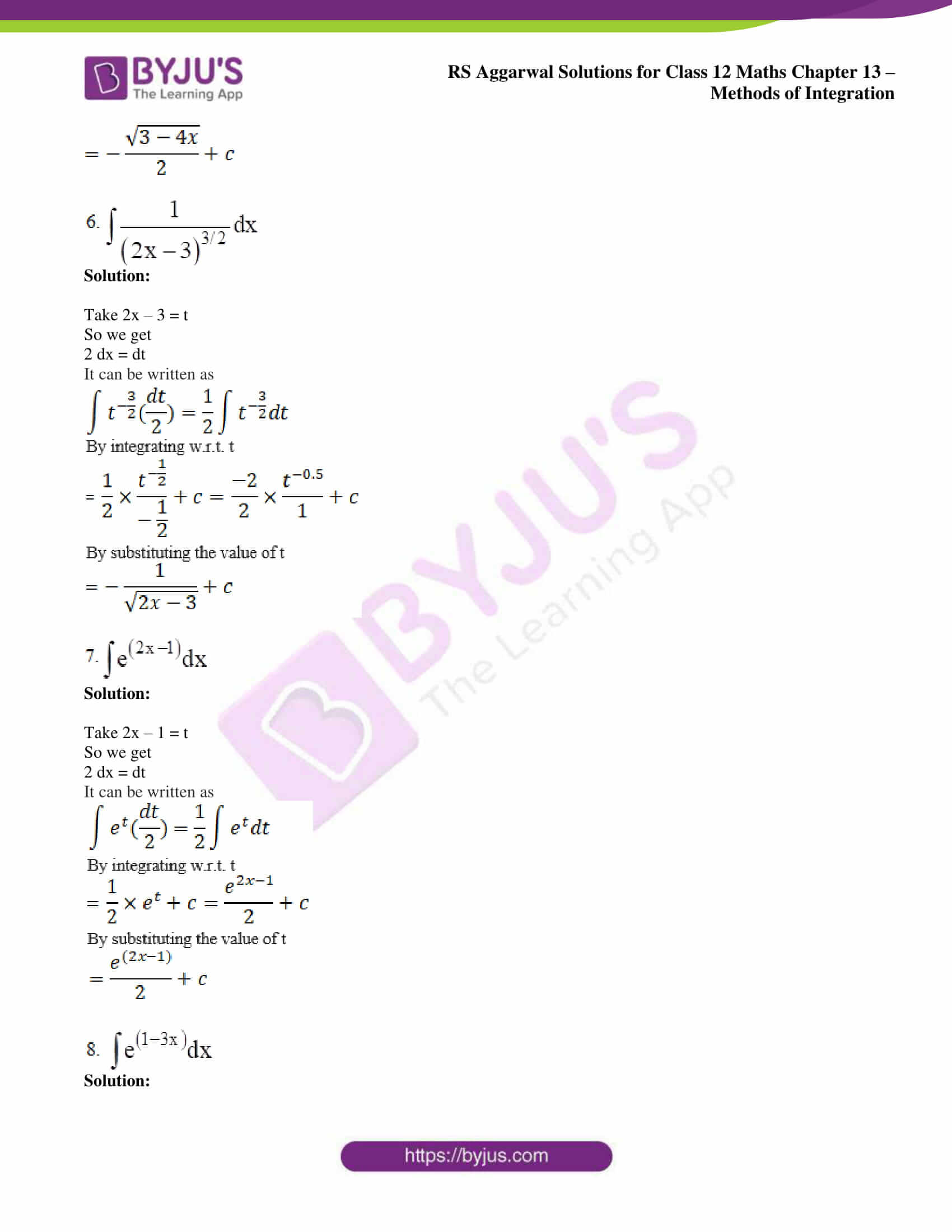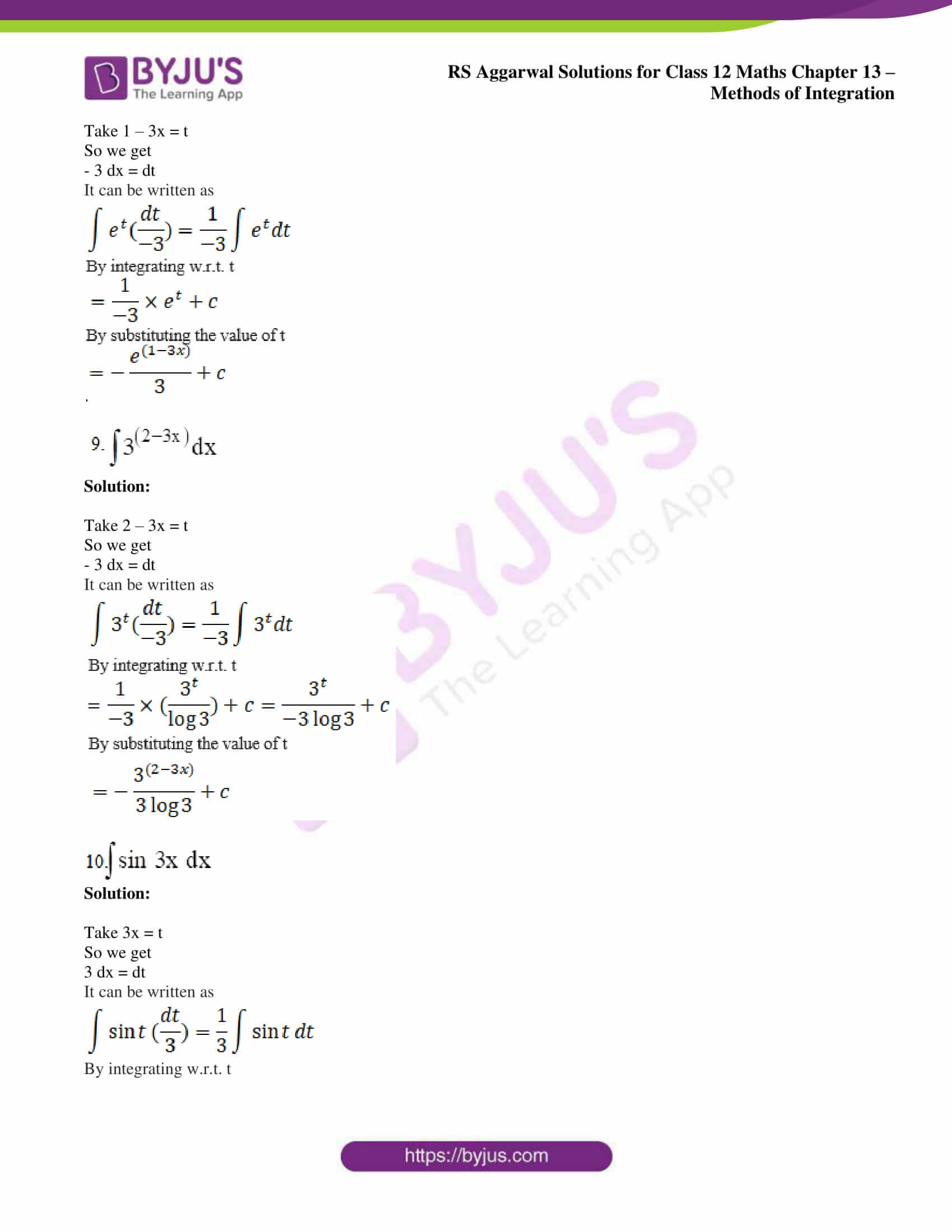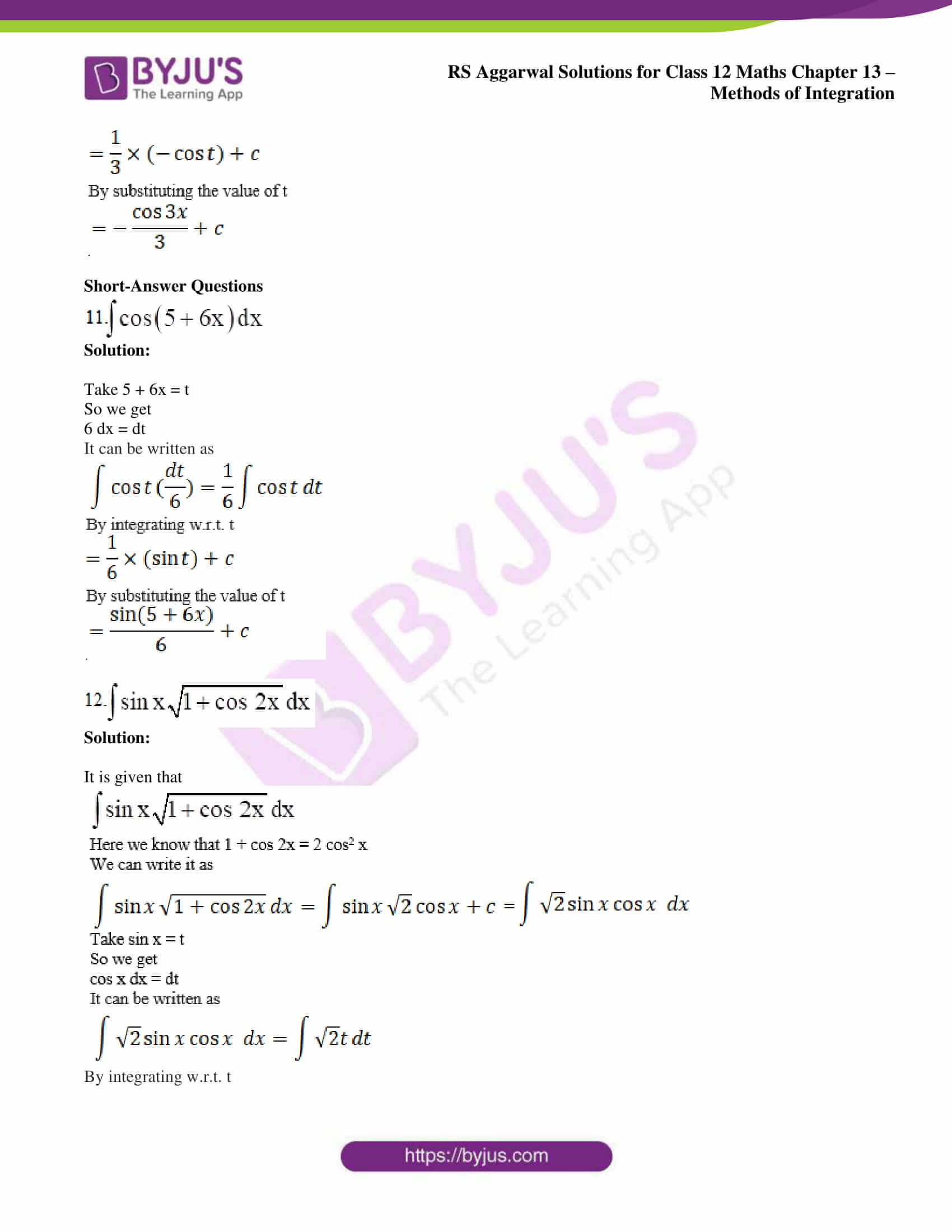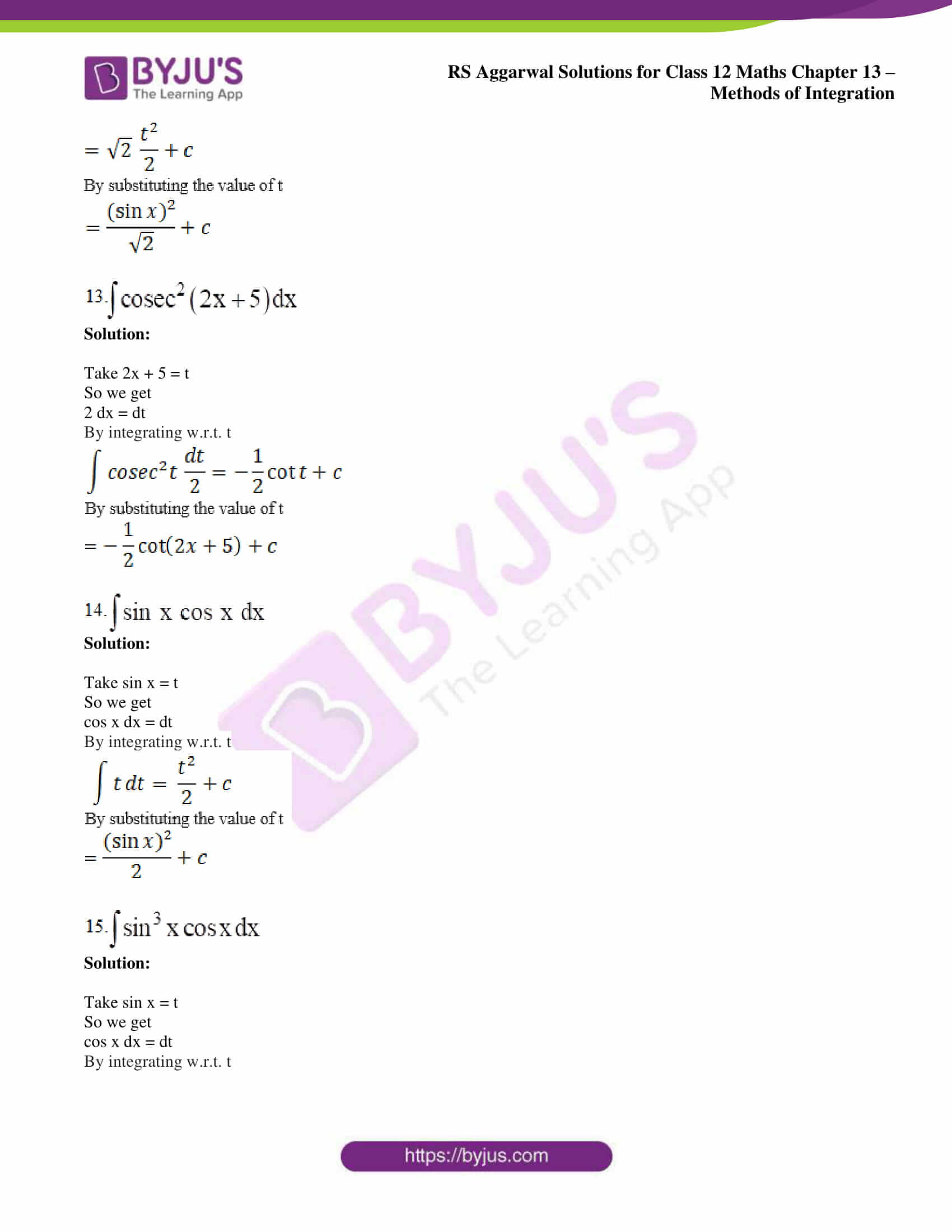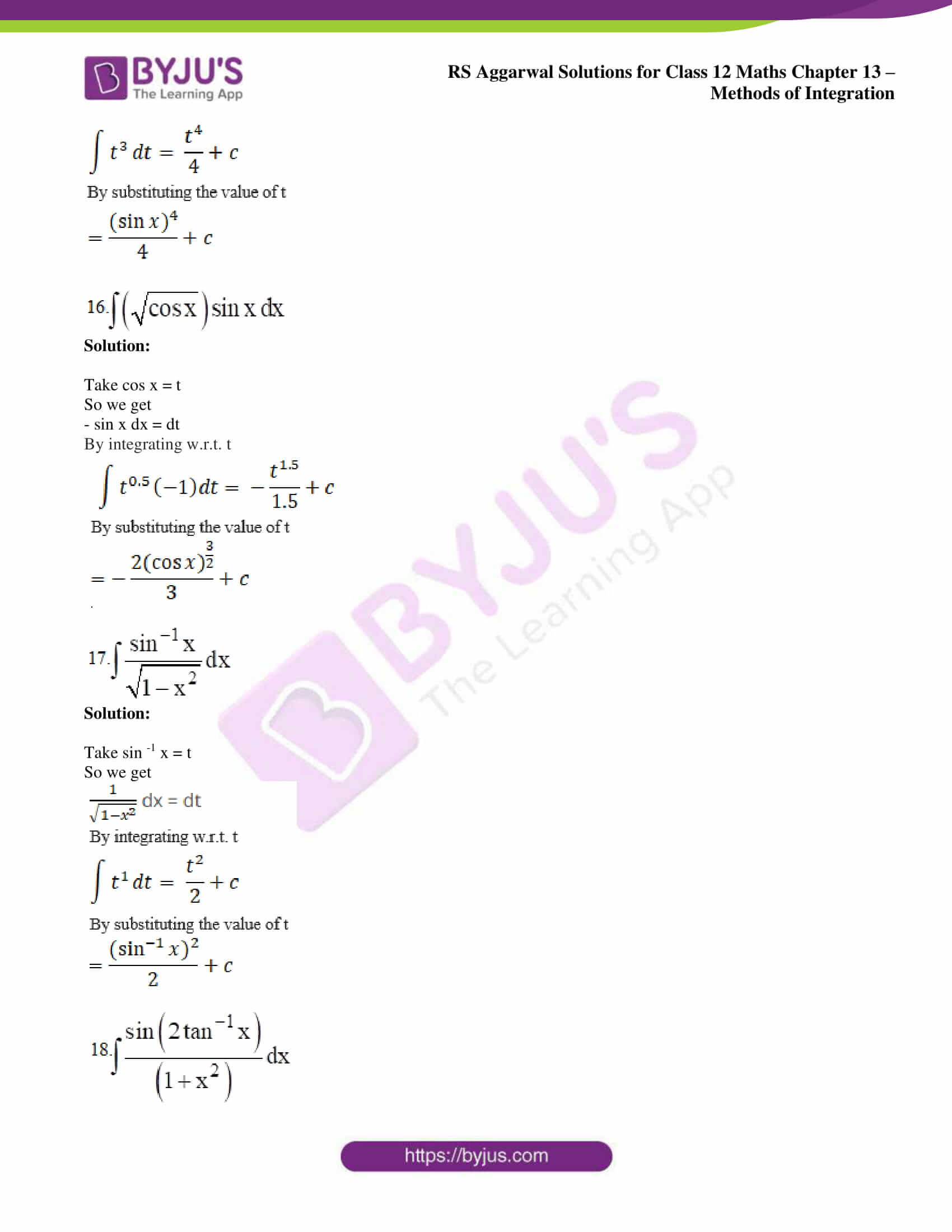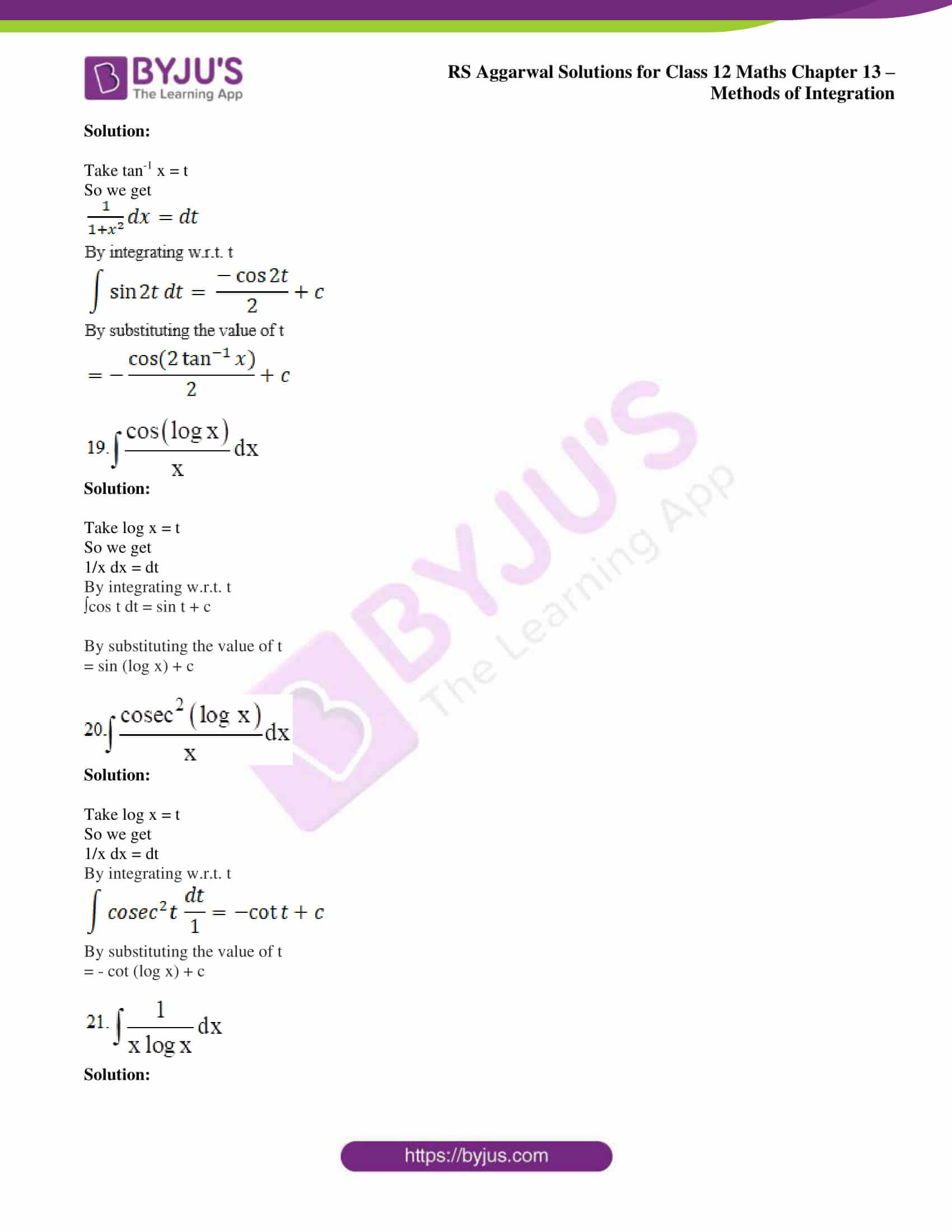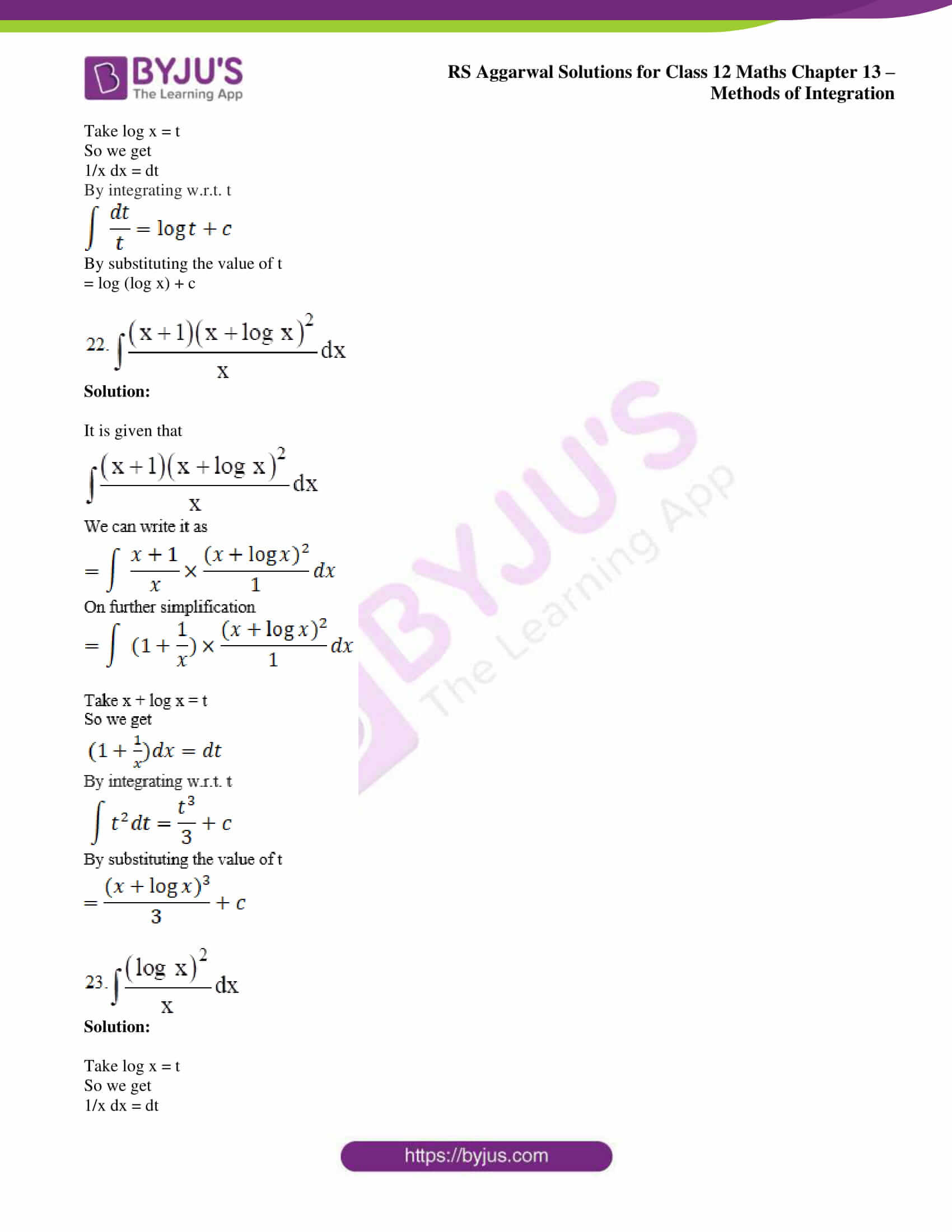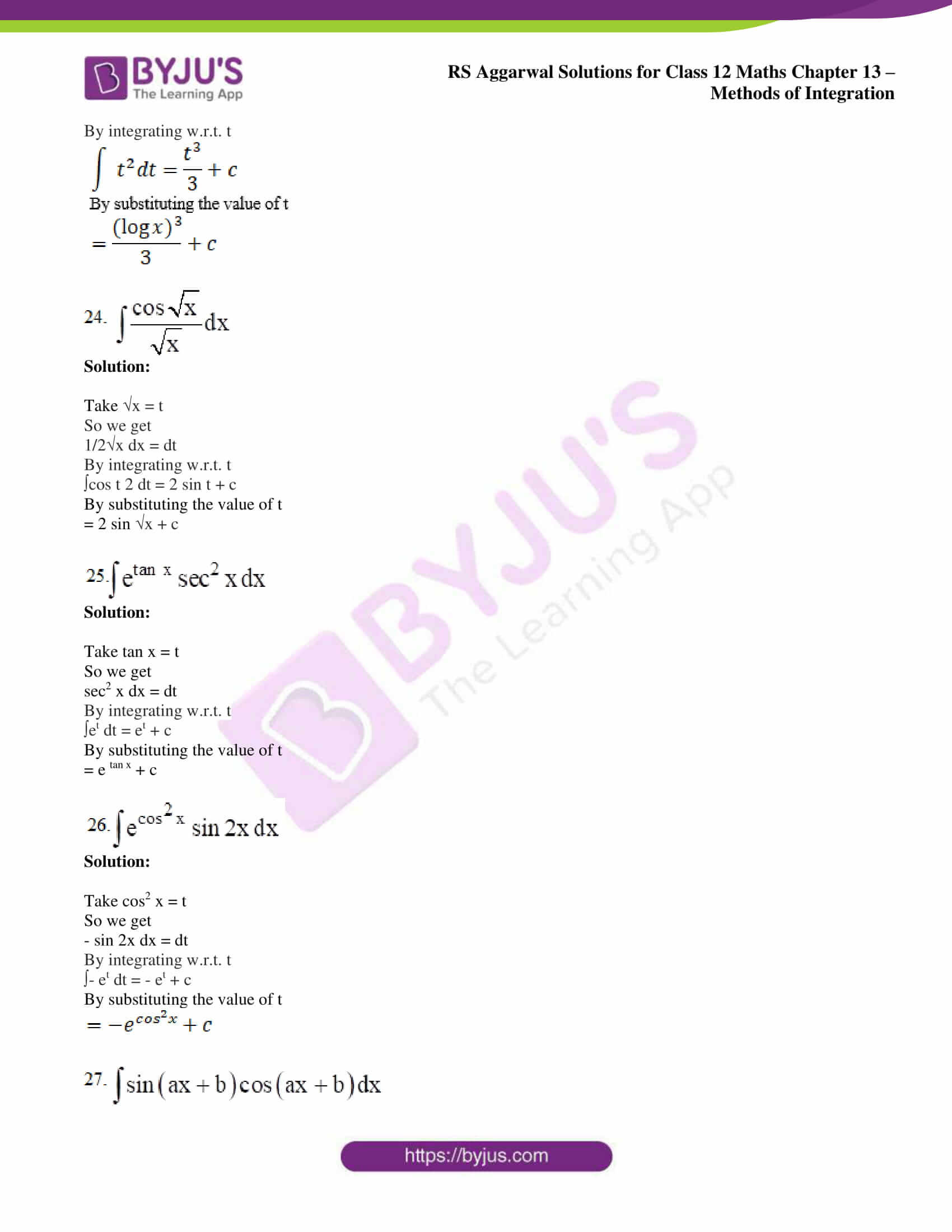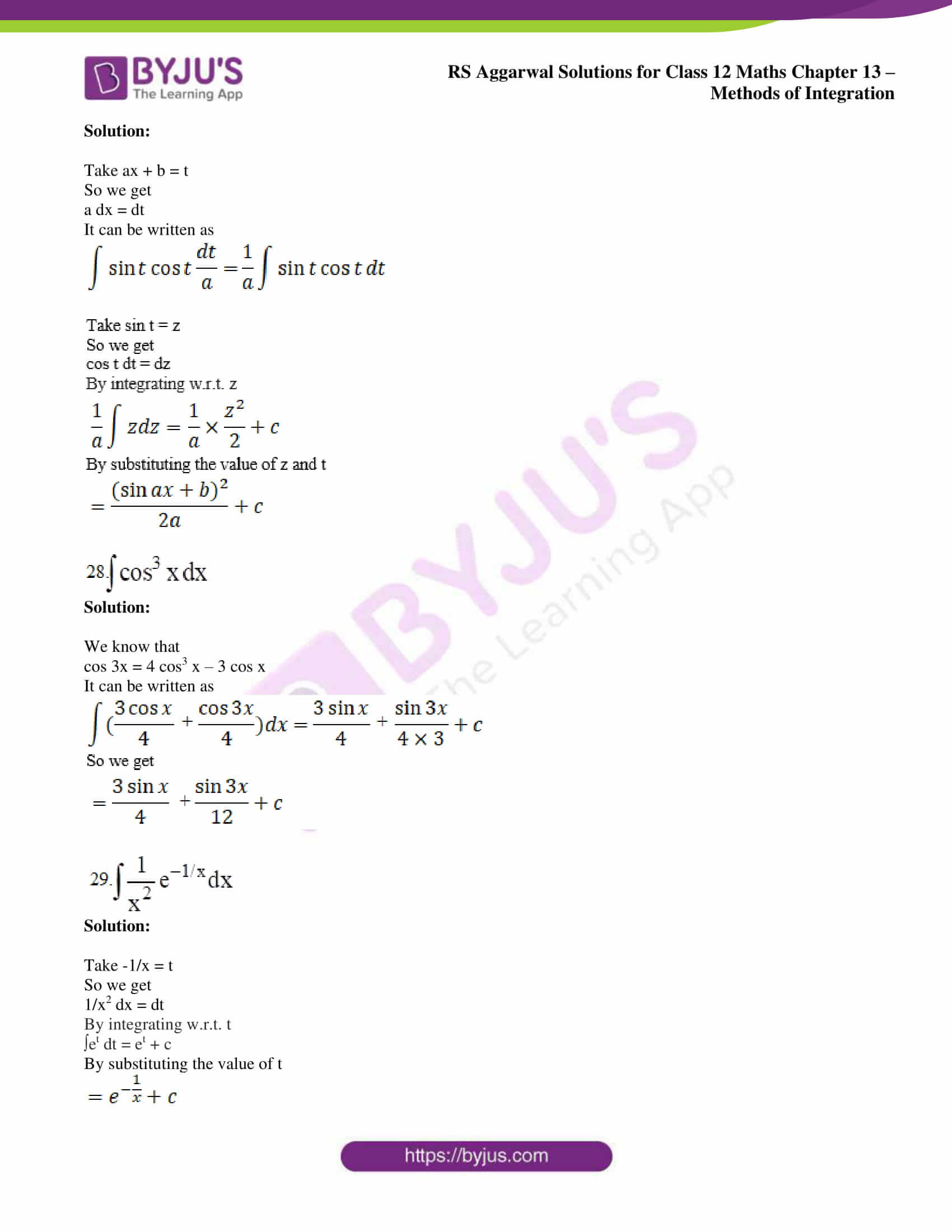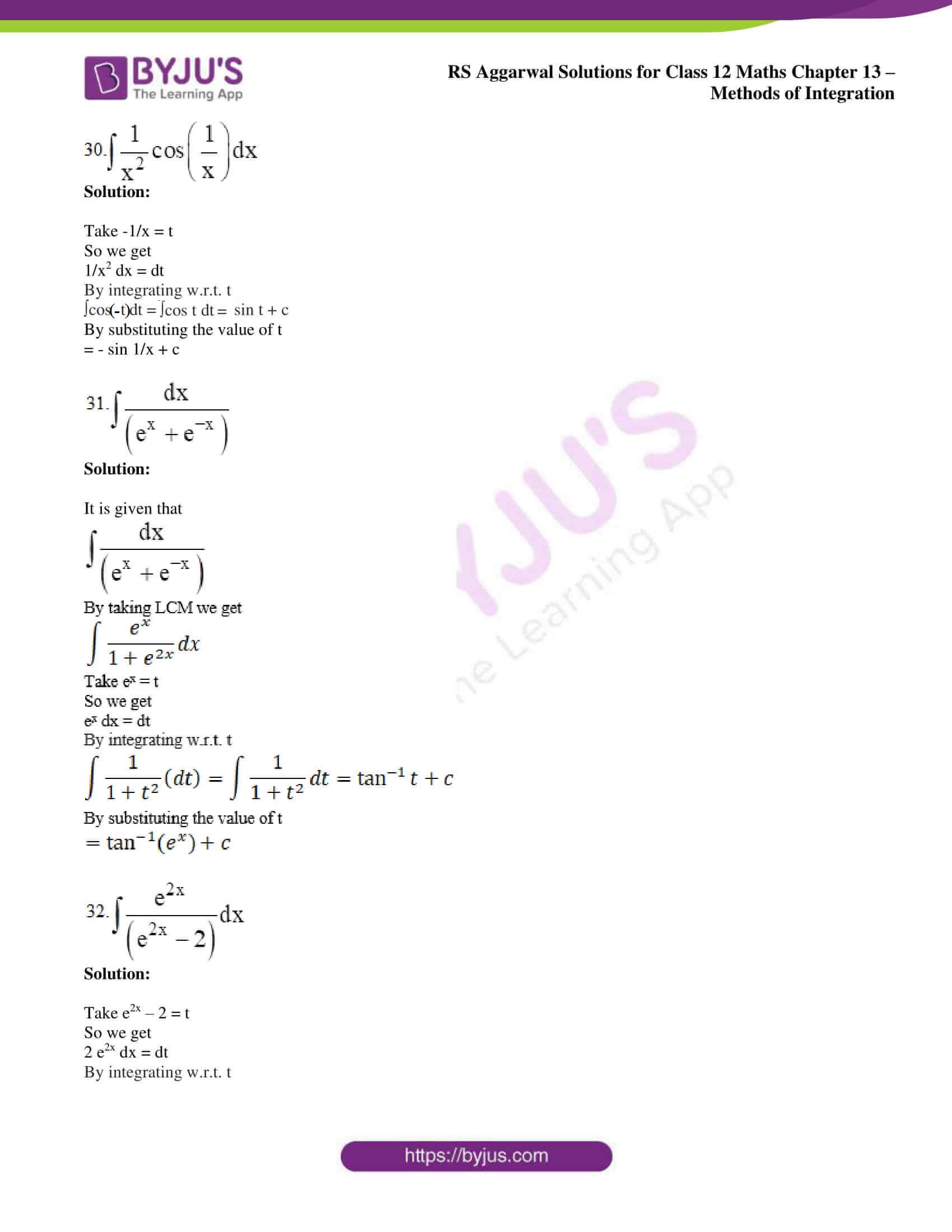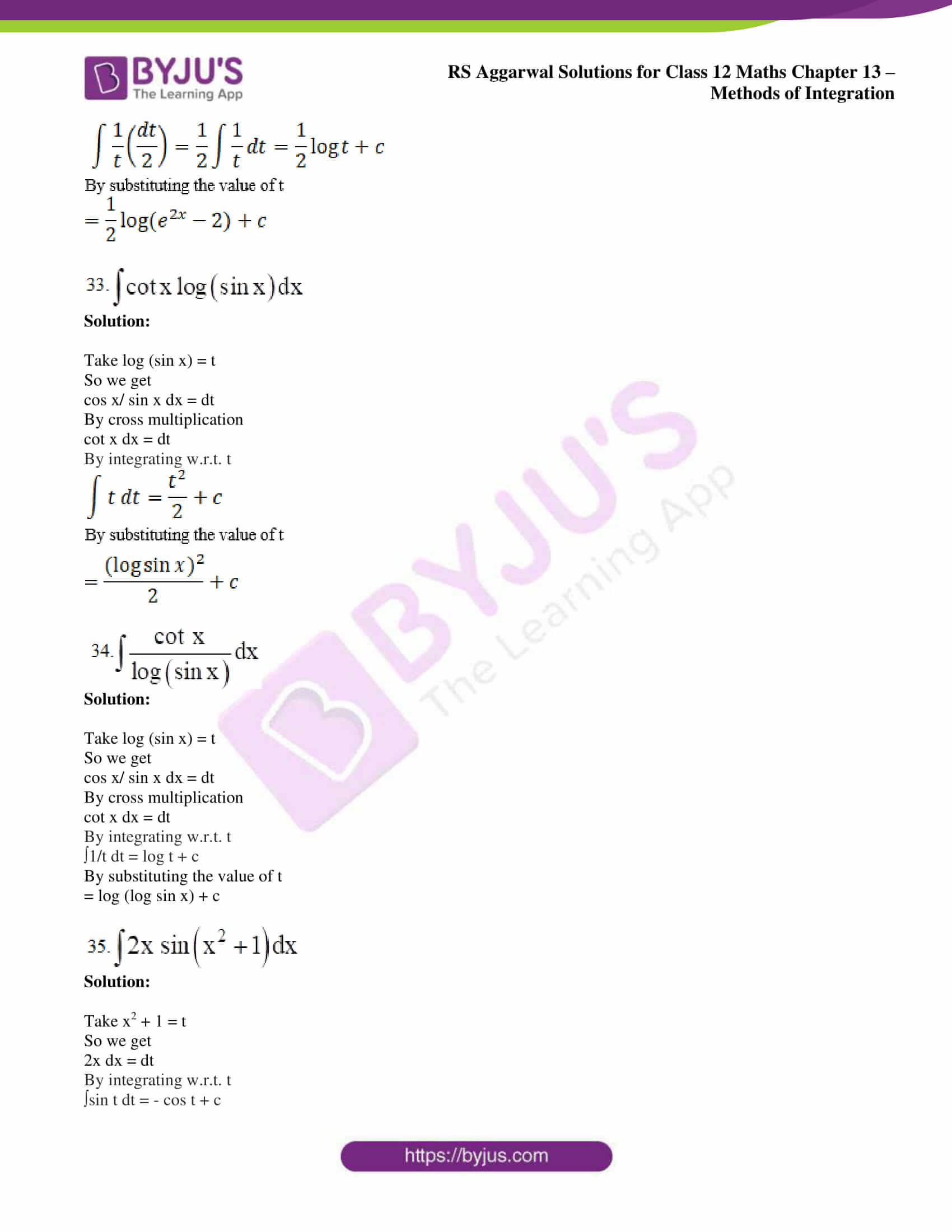### Access other exercise solutions of Class 12 Maths Chapter 13: Methods of Integration

Exercise 13B Solutions

Exercise 13C Solutions

### Access RS Aggarwal Solutions for Class 12 Maths Chapter 13: Methods of Integration Exercise 13A

Evaluate the following integrals:

1. ∫ (2x + 9) 5 dx

Solution:

Take 2x + 9 = t

So we get

2 dx = dt

It can be written as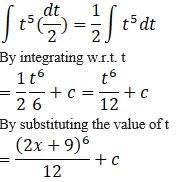2.  ∫ (7 – 3x) 4 dx

Solution:

Take 7 – 3x = t

So we get

– 3 dx = dt

It can be written as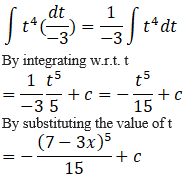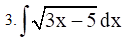Solution:

Take 3x – 5 = t

So we get

3 dx = dt

It can be written as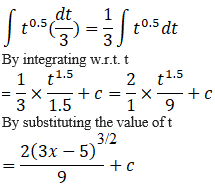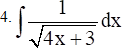Solution:

Take 4x + 3 = t

So we get

4 dx = dt

It can be written as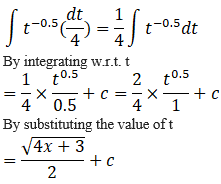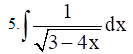Solution:

Take 3 – 4x = t

So we get

– 4 dx = dt

It can be written as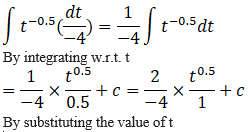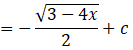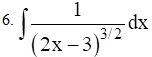Solution:

Take 2x – 3 = t

So we get

2 dx = dt

It can be written as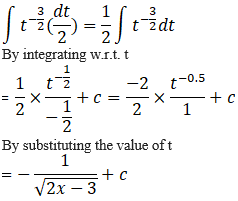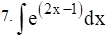Solution:

Take 2x – 1 = t

So we get

2 dx = dt

It can be written as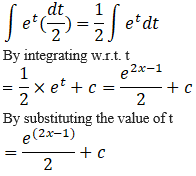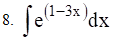Solution:

Take 1 – 3x = t

So we get

– 3 dx = dt

It can be written as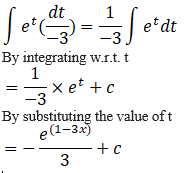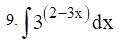Solution:

Take 2 – 3x = t

So we get

– 3 dx = dt

It can be written as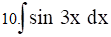Solution:

Take 3x = t

So we get

3 dx = dt

It can be written as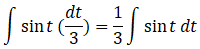By integrating w.r.t. t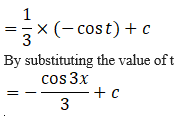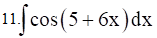Solution:

Take 5 + 6x = t

So we get

6 dx = dt

It can be written as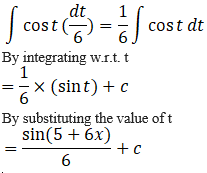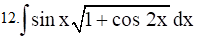Solution:

It is given that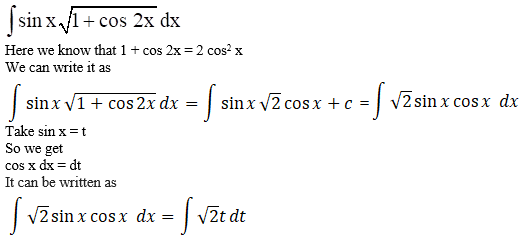By integrating w.r.t. t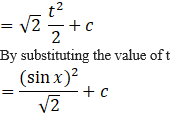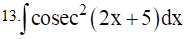Solution:

Take 2x + 5 = t

So we get

2 dx = dt

By integrating w.r.t. t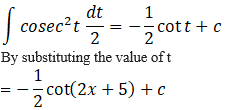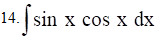Solution:

Take sin x = t

So we get

cos x dx = dt

By integrating w.r.t. t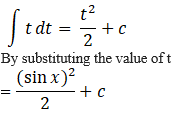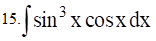Solution:

Take sin x = t

So we get

cos x dx = dt

By integrating w.r.t. t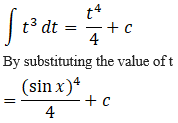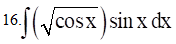Solution:

Take cos x = t

So we get

– sin x dx = dt

By integrating w.r.t. t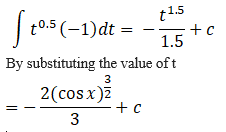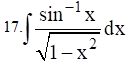Solution:

Take sin -1 x = t

So we get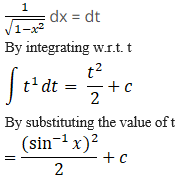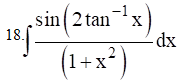Solution:

Take tan-1 x = t

So we get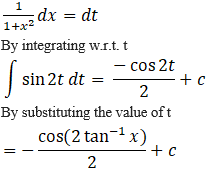Solution:

Take log x = t

So we get

1/x dx = dt

By integrating w.r.t. t

∫cos t dt = sin t + c

By substituting the value of t

= sin (log x) + c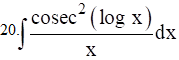Solution:

Take log x = t

So we get

1/x dx = dt

By integrating w.r.t. t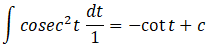By substituting the value of t

= – cot (log x) + c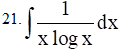Solution:

Take log x = t

So we get

1/x dx = dt

By integrating w.r.t. t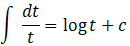By substituting the value of t

= log (log x) + c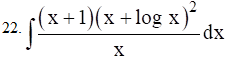Solution:

It is given that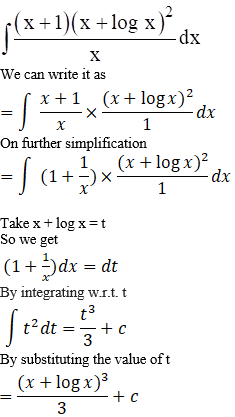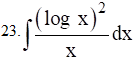Solution:

Take log x = t

So we get

1/x dx = dt

By integrating w.r.t. t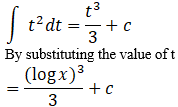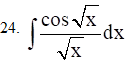Solution:

Take √x = t

So we get

1/2√x dx = dt

By integrating w.r.t. t

∫cos t 2 dt = 2 sin t + c

By substituting the value of t

= 2 sin √x + c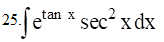Solution:

Take tan x = t

So we get

sec2 x dx = dt

By integrating w.r.t. t

∫et dt = et + c

By substituting the value of t

= e tan x + c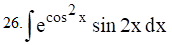Solution:

Take cos2 x = t

So we get

– sin 2x dx = dt

By integrating w.r.t. t

∫- et dt = – et + c

By substituting the value of t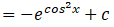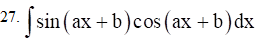Solution:

Take ax + b = t

So we get

a dx = dt

It can be written as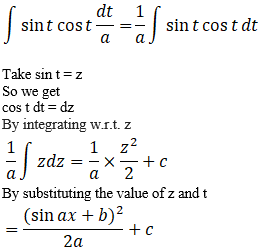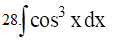Solution:

We know that

cos 3x = 4 cos3 x – 3 cos x

It can be written as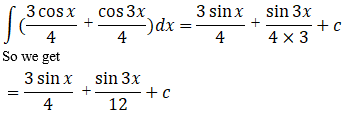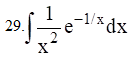Solution:

Take -1/x = t

So we get

1/x2 dx = dt

By integrating w.r.t. t

∫et dt = et + c

By substituting the value of t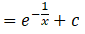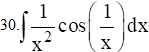Solution:

Take -1/x = t

So we get

1/x2 dx = dt

By integrating w.r.t. t

∫cos (-t) dt = ∫cos t dt = sin t + c      [Since, cos is an even function]

By substituting the value of t

= – sin 1/x + c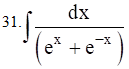Solution:

It is given that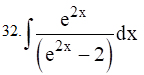Solution:

Take e2x – 2 = t

So we get

2 e2x dx = dt

By integrating w.r.t. t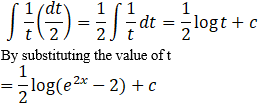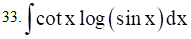Solution:

Take log (sin x) = t

So we get

cos x/ sin x dx = dt

By cross multiplication

cot x dx = dt

By integrating w.r.t. t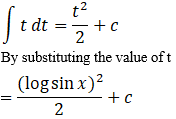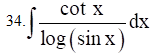Solution:

Take log (sin x) = t

So we get

cos x/ sin x dx = dt

By cross multiplication

cot x dx = dt

By integrating w.r.t. t

∫1/t dt = log t + c

By substituting the value of t

= log (log sin x) + c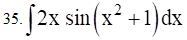Solution:

Take x2 + 1 = t

So we get

2x dx = dt

By integrating w.r.t. t

∫sin t dt = – cos t + c

By substituting the value of t

= – cos (x2 + 1) + c